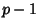## Polynomial CurveA curve obtained by fitting Polynomials to each ordinate of an ordered sequence of points. The above plots show Polynomial curves where the order of the fitting Polynomial varies fromto, whereis the number of points.

Polynomial curves have several undesirable features, including a nonintuitive variation of fitting curve with varying Coefficients, and numerical instability for high orders. Splines such as the Bézier Curve are therefore used more commonly.